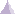# Shell PropertyShell property is a physical property assigned to surface elements.

A shell property references a material assigned to the surface part and describes a thickness associated to this surface part. A shell property is associative to the geometry this property points at.

The input and output characteristics are:

• Input:

• Material

• Thickness

• Output:

• Stress

• Strain

• Point force vector

• Point moment vector
• Stress Von Mises
• Elastic energy
• Elastic energy density
• Estimated error
• Curvature
• Transverse shear strain
• Transverse shear stress

Those characteristics can be expressed at the given positions in the elements and in different axis systems:

 Position Axis System Characteristics Center of element Nodes of element Gauss point Global Local StressStrainPoint force vectorPoint moment vectorStress Von MisesElastic energyElastic energy densityEstimated errorCurvatureTransverse shear strainTransverse shear stress